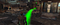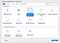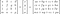# Getting Started with ARKit: WaypointsExample of a waypoint in Fallout 4

# ARKit FundamentalsStarting an Augmented Reality app is super easy

## SessionConfiguration

`configuration.worldAlignment = .gravityAndHeading`
`override func viewWillAppear(_ animated: Bool) {        super.viewWillAppear(animated)  // Create a session configuration  let configuration = ARWorldTrackingSessionConfiguration()  configuration.worldAlignment = .gravityAndHeading  // Run the view's session  sceneView.session.run(configuration)}`

`override func touchesBegan(_ touches: Set<UITouch>, with event: UIEvent?) {  guard let sceneView = self.view as? ARSKView else {    return  }  // Create anchor using the camera’s current position  if let currentFrame = sceneView.session.currentFrame {    // Create a transform with a translation of 0.2 meters in front         // of the camera    var translation = matrix_identity_float4x4    translation.columns.3.z = -0.2    let transform = simd_mul(      currentFrame.camera.transform,       translation    )    // Add a new anchor to the session    let anchor = ARAnchor(transform: transform)    sceneView.session.add(anchor: anchor)  }}`
`func view(_ view: ARSKView, nodeFor anchor: ARAnchor) -> SKNode? {  // Create and configure a node for the anchor added to the view's      // session.  let labelNode = SKLabelNode(text: "👾")  labelNode.horizontalAlignmentMode = .center  labelNode.verticalAlignmentMode = .center  return labelNode;}`

## Linear AlgebraMultiplying a 4x4 matrix by vertex results in transformed vertex
`[1 0 0 X]   [x]   [ x + X*w ][0 1 0 Y] x [y] = [ y + Y*w ][0 0 1 Z]   [z]   [ z + Z*w ][0 0 0 1]   [w]   [ W       ]`
`Translated = T x C`
`Rotated = Ry x C`
`Final Transform = T x R x S x C`
`simd_mul(C, simd_mul(R, T)) = Final Transform`

# Putting it together

`{ North: z-, East: x+, South: z+, West: x- }`
`/**Precise bearing between two points.*/static func bearingBetween(startLocation: CLLocation, endLocation: CLLocation) -> Float {  var azimuth: Float = 0  let lat1 = GLKMathDegreesToRadians(    Float(startLocation.coordinate.latitude)  )  let lon1 = GLKMathDegreesToRadians(    Float(startLocation.coordinate.longitude)  )  let lat2 = GLKMathDegreesToRadians(    Float(endLocation.coordinate.latitude)  )  let lon2 = GLKMathDegreesToRadians(    Float(endLocation.coordinate.longitude)  )  let dLon = lon2 - lon1  let y = sin(dLon) * cos(lat2)  let x = cos(lat1) * sin(lat2) - sin(lat1) * cos(lat2) * cos(dLon)  let radiansBearing = atan2(y, x)  azimuth = GLKMathRadiansToDegrees(Float(radiansBearing))  if(azimuth < 0) { azimuth += 360 }  return azimuth}`
`GLKMatrix4RotateY(GLKMatrix4Identity, theta)`
`func getTransformGiven(currentLocation: CLLocation) -> matrix_float4x4 {  let bearing = bearingBetween(    startLocation: currentLocation,     endLocation: location  )  let distance = 5  let originTransform = matrix_identity_float4x4  // Create a transform with a translation of 5meter away  let translationMatrix = MatrixHelper.translate(    x: 0,     y: 0,     z: distance * -1  )  // Rotation matrix theta degrees  let rotationMatrix = MatrixHelper.rotateAboutY(    degrees: bearing * -1  )  var transformMatrix = simd_mul(rotationMatrix, translationMatrix)    return simd_mul(originTransform, transformMatrix)}`

# Feedback/Suggestions

Written by

## Yat Choi

#### Traveller // Engineer at Airbnb // Amateur Music Producer Follow me on IG: @ yatshitcray or email me at yatchoi1@gmail.com

Welcome to a place where words matter. On Medium, smart voices and original ideas take center stage - with no ads in sight. Watch
Follow all the topics you care about, and we’ll deliver the best stories for you to your homepage and inbox. Explore
Get unlimited access to the best stories on Medium — and support writers while you’re at it. Just \$5/month. Upgrade